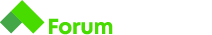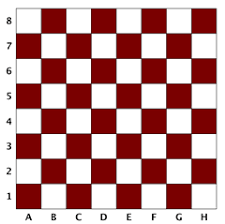# Chess Square Color

Здравейте. Не знам защо Judge системата ми дава само 60/100 за “dark” и 40/100 за “light”.
Ето го условието и след това моето решение :

# Chess Square Color

Write a program that determines the color of a chessboard square based on its Label and Rank

• Labels have values from `a` to `h`
• Ranks have values from `1` to `8`## Input

• On the first line, you will receive L - the label
• On the second line, you will receive R - the rank

## Output

• On the only line of output, print `light` or `dark` , based on your calculations

## Constraints

• `a` <= L <= `h`
• `1` <= R <= `8`

## Input

``````a
1
``````

## Output

``````dark
``````

let print = this.print || console.log;

// Use gets() to receive one line of input (the input line is always a string)
// Use “print” instead of “console.log” to print the result
let inputOne = “a”;
let inputTwo = 1;
let label = gets();
let rank = +gets();
if (label === “a” ||label === “c” ||label === “e” ||label === “g”) {
console.log(“dark”);
} else if (label === “b” || label === “d” || label === “f” || label === “h”) {
console.log(“light”) ;
}
if ( rank === 1 || rank === 3 || rank === 5 || rank === 7) {
console.log(“dark”) ;
} else if ( rank === 2 || rank === 4 || rank === 6 || rank === 8) {
console.log(“light”) ;
}
print(“dark”);

Преместих поста ти в Alpha Preparation tasks. Когато няма категория, по-малко хора го виждат.